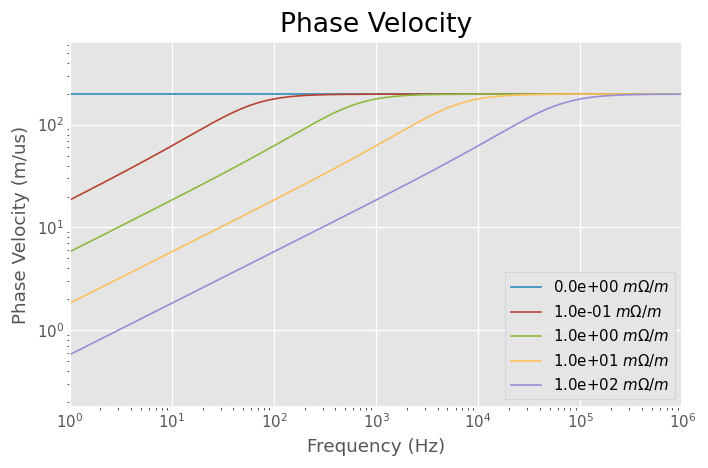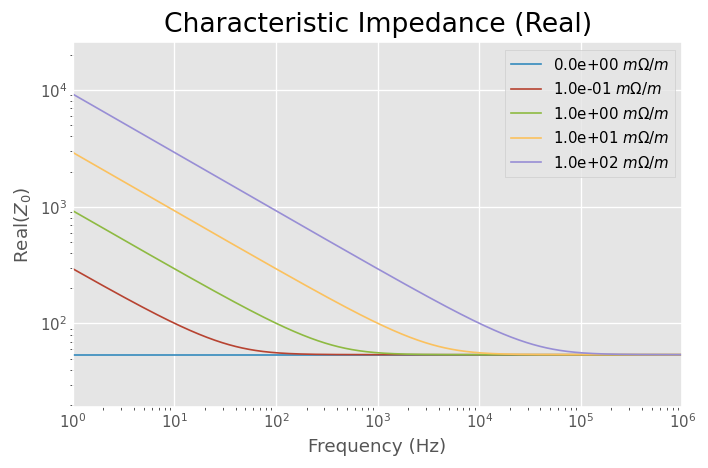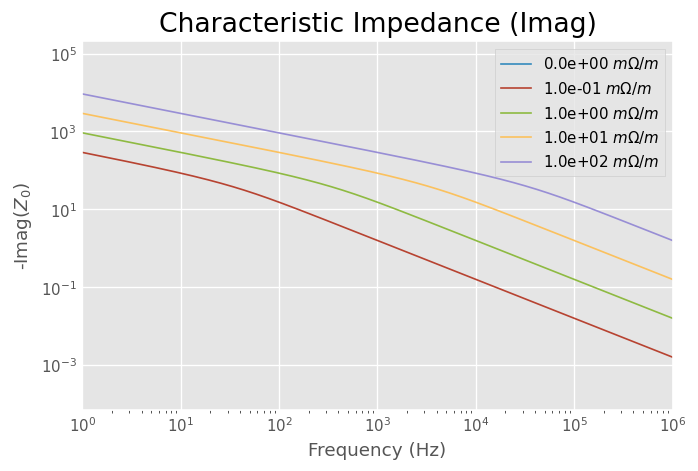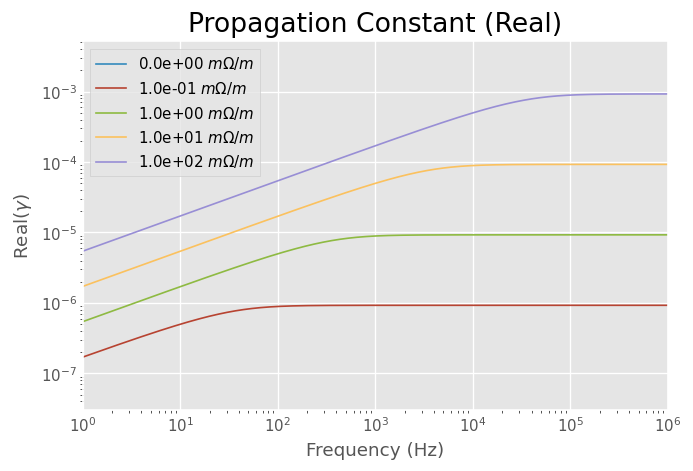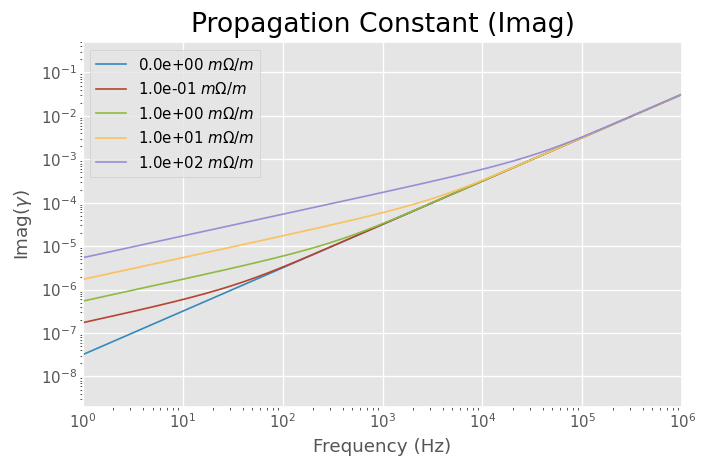# Properties of RG-58¶

This example demonstrates how to use skrf’s to explore some properties of Coax (RG-58). This is done through the media package which provides basic transmission line models. Specifically, the coax is created using by DistributedCircuit.

:

import skrf as rf
%matplotlib inline
from pylab import *
rf.stylely()

# define a frequency object from a vector
freq = rf.F.from_f(logspace(0,6,1001), unit='hz')

# create a Media object for RG-58, based on distributed ckt values
rg58 = rf.media.DistributedCircuit(
frequency = freq,
C =93.5e-12,#F/m
L =273e-9,  #H/m
R =0,#53e-3,   #Ohm/m
G =0,       #S/m
)

# loop thru values of resistivity and plot various quantities
for k in (0,.1,1,10,100):
rg58.R = k*1e-3

figure(0)
ylabel('Phase Velocity (m/us)')
title('Phase Velocity')
loglog(freq.f_scaled, rg58.v_p*1e-6, label=r' %1.e $m \Omega/m$'%k)

figure(1)
ylabel('Real($Z_0$)')
title('Characteristic Impedance (Real)')
loglog(freq.f_scaled, rg58.z0.real, label=r'%1.e $m \Omega/m$'%k)

figure(2)
ylabel('-Imag($Z_0$)')
title('Characteristic Impedance (Imag)')
try:
plot(freq.f_scaled, -1*rg58.z0.imag, label=r'%1.e $m \Omega/m$'%k)
except:
pass

figure(3)
ylabel('Real($\gamma$)')
title('Propagation Constant (Real)')
try:
plot(freq.f_scaled, rg58.alpha, label=r'%1.e $m \Omega/m$'%k)
except:
pass

figure(4)
ylabel('Imag($\gamma$)')
title('Propagation Constant (Imag)')
try:
plot(freq.f_scaled, rg58.beta, label=r'%1.e $m \Omega/m$'%k)
except:
pass

from skrf.plotting import func_on_all_figs as foaf

foaf(freq.labelXAxis)
foaf(tight_layout)
foaf(legend)
foaf(loglog)

tight_layout()


/home/docs/checkouts/readthedocs.org/user_builds/scikit-rf/conda/latest/lib/python3.5/site-packages/matplotlib/style/core.py:51: UserWarning: Style includes a parameter, 'interactive', that is not related to style.  Ignoring
"to style.  Ignoring".format(key))
/home/docs/checkouts/readthedocs.org/user_builds/scikit-rf/conda/latest/lib/python3.5/site-packages/numpy/core/numeric.py:501: ComplexWarning: Casting complex values to real discards the imaginary part
return array(a, dtype, copy=False, order=order)